### 12.1 Synopsis

The LinearElasticSolver is a solver for solving the linear elasticity equations in two and three dimensions. Whilst this may be suitable for simple solid mechanics problems, its main purpose is for use for mesh deformation and high-order mesh generation, whereby the finite element mesh is treated as a solid body, and the deformation is applied at the boundary in order to curve the interior of the mesh.

Currently the following equation systems are supported:

 Value Description `LinearElasticSystem` Solves the linear elastic equations. `IterativeElasticSystem` A multi-step variant of the elasticity solver, which breaks a given deformation into multiple steps, and applies the deformation to a mesh.

#### 12.1.1 The linear elasticity equations

The linear elasticity equations model how a solid body deforms under the application of a ‘small’ deformation or strain. The formulation starts with the equilibrium of forces represented by the equation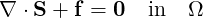(12.1)

where S is the stress tensor and f denotes a spatially-varying force. We further assume that the stress tensor S incorporates elastic and, optionally, thermal stresses that can be switched on to assist in mesh deformation applications. We assume these can be decomposed so that S is written as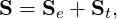where the subscripts e and t denote the elastic and thermal terms respectively. We adopt the usual linear form of the elastic stress tensor as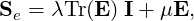where λ and μ are the Lamé constants, E represents the strain tensor, and I is the identity tensor. For small deformations, the strain tensor E is given as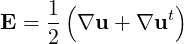(12.2)

where u is the two- or three-dimensional vector of displacements. The boundary conditions required to close the problem consist of prescribed displacements at the boundary ∂Ω, i.e.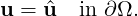(12.3)

We further express the Lamé constants in terms of the Young’s modulus E and Poisson ratio ν as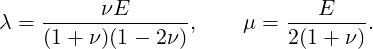The Poisson ratio, valid in the range ν <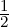, is a measure of the compressibility of the body, and the Young’s modulus E > 0 is a measure of its stiffness.